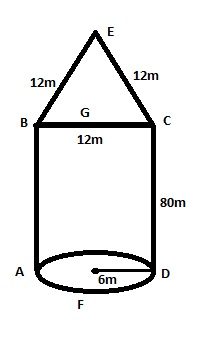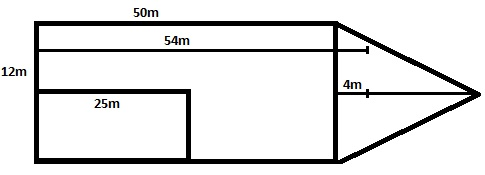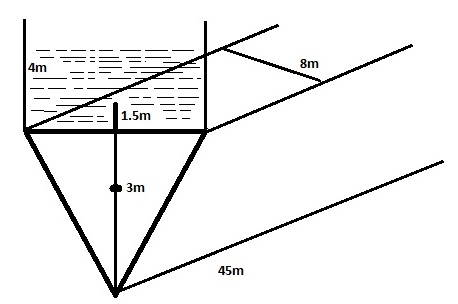Stability – I : Chapter 8 |
Miscelleneous

# Stability – I : Chapter 8

###### Solution:

Displacement = 1640

Volume of box shape vessel = (L x B x H)
= (50m x10m x 8m)

RD = 1.025

Displacement = (u/w volume) x(density of water displaced)
1640         = ( L x B x d )  x (1.025)
1640              =(50 x 10 x d) x (1.025 )

Hence, d =   1640/ (50 x 10x 1.025)
= 3.2m

As we know that  KB can be calculated as
= (d/2)
= (3.2/2)
= 1.6m

###### Solution :

Volume of box shape vessel = ( L X B XH)
=(60m x 10m x 10m)

RD = 1.020,
Draft = 6m

Displacement at RD of 1.020 = (u/w volume)x(density)
=    ( L x B x d ) x 1.020
= (60 x 10 x 6) x 1.020
= 3672t

Now we have to calculate draft of vessel at RD of 1.004,
keeping the displacement same    = (L x B x d ) x (1.004)
3672 = (60 x 10 x d )x (1.004)
d = 3672 /(60 x 10 x 1.004)
= 6.095m

Hence,     KB = (d/2
= (6.095 /2)
= 3.047m.

###### Solution:

Displacement = 650t,
RD = 1.95

Water plane Area =(L x B)
= (30m x 8m)

We know that:
Volume of triangular vessel =   ½ ( L  x B x  H)

Displacement = (u/w volume)x (Density)
650 =  ½ (L x B x  d ) x( density)
=(½ x 30 x 8 x d) x(1.015)
d = (650 x 2)/ (30 x 8 x 1.015)
= 5.336m

As we know that centeriod of triangle is 2/3rd of the perpendicular bisector of the base .Hence centre of buoyancy will be always 2/3rd of perpendicular bisector of base.

KB =( 2/3 x height of  perpendicular bisector of base)
= (2/3 x 5.336)
= 3.557m.

###### Solution :

Water plane area = (L x B)
= 40m x 12m

Initial KB =3.6m,

As we know that centeriod of triangle is 2/3rd of the perpendicular bisector of the base .hence centre of buoyancy will be always 2/3rd of perpendicular bisector of base.

So, KB = 2/3 x (Draft)
3.6    = 2/3 x d

So, Draft(d)  =(3.6 x 3 / 2)
= 5.4m

We know that:
Volume of triangular vessel =   ½ ( L  x B x  H)

Displacement =(u/w volume) x (density)
= ½  (L x B x d) x (1.025)
= 1/2  (40 x 12 x 5.4) x (1.025
= 1328.4t .

###### Solution:

Volume of log of wood = (L x B x H)
= (3m x 0.75m x 0.75m)

RD of SW = 1.025
RD of the log = 0.8m

Mass of the log = (volume x density)
= (3 x 0.75 x 0.75 ) x (0.8)
= 1.35 t

Since,  log floats freely so
Mass = Displacement
1.35 = (u/w volume) x(density)
1.35 = (L x B x draft) x  (1.025)
1.35 = ( 3x 0.75 x draft) x (1.025)

So, draft = 1.35/(3 x 0.75 x 1.025)
Draft  = 0.585 m

Again, we know that:
COB = (1/2 x draft)
= (0.585/2)
= 0.2925m

COG = (1/2 x height)
= (0.75 / 2)
= 0.375m

Hence, Vertical distance between COB and COG
= (0.375 – 0.295)
= 0.083m

###### Solution:

RD = 1.005 & Draft  = 0.4m

We know that:
Displacement (W) = (volume ) x (density)
= (0.5 x 0.5 x 0.4) x(1.005)
= 0.1005t

Now ,calculating draft for the same displacement  at RD of 1.020.
W = ( Area x draft ) x( density)
0.1005 =(0.5 x 0.5 x draft) x( 1.020)

Draft = 0.1005/(0.5 x 0.5 x 1.020)
= 0.394m

Again , we know that :
COB = (draft /2)
= (0.395/2)
= 0.197m

COG = (Height/2)
= (0.5/2)
= 0.25m

Now, Vertical distance between COG and COB can be easily calculated as = (0.25 – 0.197)
= 0.053m

###### Solution :

Diameter = 0.8m ,
So, radius = (0.8 /2) = 0.4m

Height = 1.5m
Weight = 10kg = (0.01) t
Mass of steel = 490kg = 0.49t
Total displacement = (0.01 + 0.49)
= 0.50t

Displacement (W) = (u/w volume) x (density)
0.5 =  r2 d) x  (RD)
0.5 = (3.1416 x 0.4 x 0.4 x d) x (1)
So, d = 0.5/(3.1416 x 0.4 x 0.4) x(1)
= 0.995m

Again, KB can be calculated as = (draft/2)
= 0.4975m

###### Solution:

According to the question the drawn figure can consist of 3 difference structure ; namely  triangle BEC , rectangle ABCD, semicircle  AFD .

Total displacement = (5000 + 500)
= 5500t

As we know that:
Total displacement = (Total u/w volume) x (density)Total u/w volume = (volume of  triangle BEC) + (Volume of rectangle ABCD) + (Volume of semicircle AFD)

Area of triangle BCE   = (π r /2)
= (3.14 x 6 x 6 )/ 2
= 56. 556m2

Area of rectangle ABCD = (L X B )
= (80 x 12)
= 960m2

Area of semicircle   AFD   = (1/2 x b x h)
= (1/2 x 12 x 10.39)
= 62.34m2

In triangle BEC,  EG is the height

So , EG2 = BE2 – BG2
EG= 122 -62

=    144 – 36
=108
EG = 10.39m

Hence, Total area = (56.55 + 62.34 + 960.0)
= 1078.89m2

Displacement (W)= (u/w volume) x (density)
5500 = (Area x depth) x (density)
5500 = (1078.89 x d ) x (1.025)

Draft = 5500 /(1078.89 x 1.025)
= 4.97m

Now, KB = (draft)/2
= (4.97/2)
= 2.485m.

###### Solution;

Total displacement = 3444tDisplacement (W) = (u/w volume ) x (density)
3444     =    (u/w volume of triangle ) + (u/w volume of rectangle )     x (1.025)
3444    =    (( 1/2 x 12 x 12 x d)  + ( 50 x 12 x d) )  x 1.025
3444   =    (72 d + 600 d) x 1.025
3444 =    (672d x 1.025)
3444 = 688.8 d

So, d = 3444/ 688.8
d =  5 m

now, COB =(draft/2)
=(5/2) m
=  2.5 m with reference to keel

Now for calculating COB with reference to after end
Total moment of barge = (moment of triangle)  + (moment of rectangle)
(3444 x LCB of barge) = ( u/w volume )x (density) x(LCB)  + (u/w volume ) x (density ) x (LCB)
(3444 x LCB)  = (1/2 x 12 x 12  x 5)  x (1.025) x( 54)  +   (50 x 12 x 5 ) x (1.005 ) x (25)
(3444 x LCB) = (19926)  + (76875)
(3444 x LCB)      = 96801
LCB = (96801 / 3444)
= 28.10m.

###### Solution:

Total displacement = 1620t

Let ‘X’  t  of weight which is  not displaced by triangleSo weight displaced  is (1620 – X )t

Area of triangle = ½ ( L x B x H)
= (1/2 x 45 x 8 x 3)
= 540m3

Displacement (W) = (u/w volume) x (density)
= 540 x 1
= 540t  = X( as per assumption made above  in solution )

Displacement of rectangle = (1620 – X)
= (1620 – 540)
= 1080t

Now ,displacement for rectangle = (u/w volume) x (density)
1080 = (45 x 8 x draft)  x (1)
Hence, draft = 1080/(45 x 8)
draft = 3 m

for calculating COB = (draft/2)
= (3/2)
= 1.5m

So, COB with respect to keel = (3.0 + 1.5)m
= 4.5m .

Now, VM ( vertical moment) created by triangle:
=(W x d)
= (540 x 2)
= 1080 tm.

Now , VM ( vertical moment) created by rectangle:
= (W x d)
= (1080 x 4.5)
= 4860 tm.

final vertical moment = ( VM) triangle  + (VM) rectangle
Final Weight = 1620t

We know that:
Final KB = (Final VM / Final W)
= (5940/ 1620)
= 3.66 m#### Vikrant_sharma

•Nitesh says:
•k shivam says:
•zeeshan Altamash says: